# Limits of Concentrations when Measuring Zeta Potential

The Zetasizer Nano series can measure zeta potential, molecular weight and particle size. The inclusion of NIBS® technology allows sizing measurements to be carried out on turbid, concentrated samples. Conversely, the concentration requirements for the measurement of the samples’ zeta potential are much lower than that for sizing measurements.

This article aims to explain the concentration limits for making successful zeta potential measurements. A case study is used to demonstrate the limits of concentration and explain what happens to the quality of the data if the concentration of the sample used is unsuitable.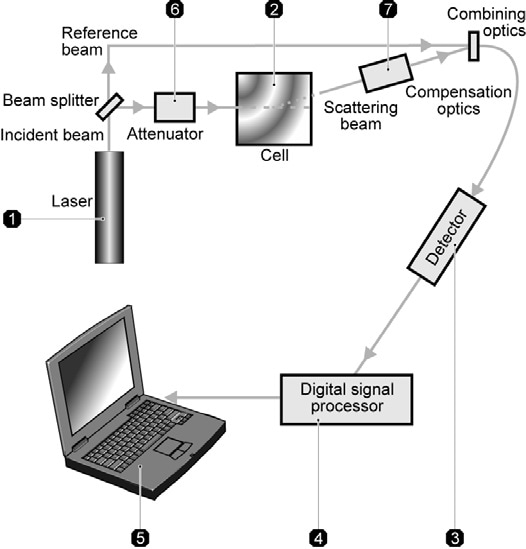Figure 1. Optical configuration of the Zetasizer for zeta potential measurement

## Optical Configuration for Zeta Potential Measurements

Figure 1 illustrates the optical configuration on the Zetasizer Nano for zeta potential measurements.

Initially, a laser ➀ is used as a light source to illuminate the particles within the sample. To measure zeta potential, this light source is split in order to offer a reference and incident beam. The intensity of the reference beam is generally between 3500 and 2000 kcps and is factory set. The incident laser beam traverses the center of the sample cell ➁, and the scattered light is detected at a forward angle ➂.

On applying an electric field across the cell, any particles traveling through the measurement volume will cause the intensity of light detected to fluctuate with a frequency dependent on the particle speed and this information is sent to a digital signal processor ➃ and PC ➄. The detected data is analyzed by the Zetasizer Nano software to obtain the electrophoretic mobility and thus zeta potential information.

This optical configuration is called heterodyne detection. In this mode of operation, the scattered light from the sample is combined with unscattered laser light (the reference beam). By using an attenuator ➅, the ratio of the intensity of the reference beam to scattered light is carefully controlled and is set to almost 10:1 in the Nano series. This attenuator changes the intensity of the laser and thus changes the intensity of the scattering. For samples that do not scatter much light like samples of low concentration or very small particles, the amount of scattered light being detected should be increased. The attenuator will thus automatically permit more light through to the sample. For samples that considerably scatter light, for example, samples at high concentration or large particles, the attenuator automatically decreases the amount of light that passes through to the sample.

In order to correct any differences in the thickness of the cell wall and the dispersant refraction, compensation optics ⑦ are set up to maintain optimum alignment.

## Measurement Protocol

When a zeta potential measurement is initiated in the Zetasizer Nano, the intensity of the reference beam is determined and displayed in the Log Sheet. The attenuator is used to adjust the intensity of the incident beam so that the intensity of the scattered light being detected is no more than 1/10th of the reference beam. For instance, if the intensity of the measured reference beam is 2600 kcps, then the attenuator will adjust until the intensity of the scattered light being detected is no greater than 260 kcps. Within the Zetasizer Nano, the attenuator has 11 positions that cover an attenuator range of 100% to 0.0003%. Table 1 shows the relationship between the attenuator position and the transmission value. The percentage of laser light that enters the sample cuvette is the transmission value.

Table 1. Relationship between the attenuator index and transmission value

Attenuator Index Transmission (% Nominal)
1 0.0003
2 0.003
3 0.01
4 0.03
5 0.1
6 0.3
7 1
8 3
9 10
10 30
11 100

The minimum count rate that is adequate for a zeta potential measurement to continue is set to 20 kcps. If the count rate detected is below this value, the measurement will terminate.

## Sample Concentration Requirements

It has been observed from the earlier discussion that the laser beam should go through the sample for scattered light at a forward angle to be detected. Thus, in general, samples for zeta potential measurements need to be optically clear. The maximum and minimum sample concentrations that can be measured will depend on the following factors:

• Particle size
• Polydispersity of the particle size distribution
• Optical properties of the particles

## Minimum Concentration Required for Zeta Potential Measurements

The minimum count rate of scattered light needed to make a measurement is 20 kcps. Hence, the minimum concentration needed will depend on the particle size and the relative refractive index (the difference in refractive index properties of the particle and the medium). As the particle size increases, it produces more scattered light and hence the concentration that can be measured is lower. For instance, consider a dispersion of a ceramic powder such as titania which has a particle refractive index of about 2.5. This relative refractive index leads to a very high level of scattering. As a result, the minimum concentration of titania, with a mean particle size of approximately 300 nm that can be measured, could be as low as 10-6% w/v.

In case the relative refractive index becomes lower, such as with proteins, for instance, the minimum concentration will be much higher. A minimum concentration of between 0.1 and 1% w/v may be needed to obtain adequate scattering from a protein solution to make a successful zeta potential measurement.

Eventually, the minimum concentration needed for successful zeta potential measurements of a particular sample has to be determined experimentally.

## Maximum Concentration Required for Zeta Potential Measurements

What is the maximum concentration that can be measured during a zeta potential measurement in the Zetasizer Nano? This question has no simple answer. All the mentioned factors have to be considered, that is, the particle size and polydispersity and the optical properties of the sample.

The scattered light from a sample in a zeta potential measurement is detected at a forward angle in the Zetasizer Nano. Hence, the laser beam needs to penetrate through the sample. If the sample concentration becomes too high, the laser beam will become attenuated by the particles decreasing the scattered light that is being detected. To try and offset these effects, the attenuator position in the instrument will be adjusted to a higher index, that is, a higher transmission.

## Case Study: Intralipid

Results are now shown in a case study to try and demonstrate the concentration limits for successful zeta potential measurements. The results obtained from a series of zeta potential measurements on a sample of Intralipid are illustrated in Figure 2. This is a commercial oil in water emulsion sample with a mean size of about 220 nm. Figure 3 shows the intensity size distribution from a dynamic light scattering measurement obtained at 0.01% w/v concentration. All zeta potential measurements were performed in the disposable zeta cell (DTS1060) using the General Purpose protocol at 25 oC. A manual duration of 40 sub runs was used.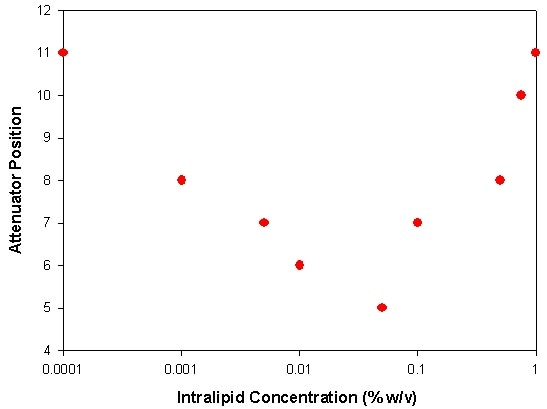Figure 2. A plot of the attenuator position automatically selected by the Zetasizer Nano at various Intralipid concentrations diluted in DI water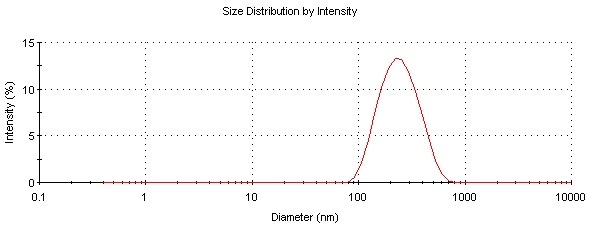Figure 3. Intensity size distribution of Intralipid measured at a concentration of 0.01% w/v

The sample at low concentrations (0.0001% w/v) scatters low levels of light and thus the attenuator adjusts to position 11 (100% transmission). As the concentration increases, more scattering is detected and therefore to make sure that the ratio of scattered light to reference beam intensity is appropriate, a lower attenuator position is chosen (lower transmission). This effect continues until a concentration of 0.05% w/v is reached at which an attenuator position 5 is used for the measurements (0.1% transmission).

At above 0.05% concentrations, the laser beam becomes attenuated by the large numbers of particles in its path and hence the attenuator position chosen now starts to increase to make sure that adequate scattering is being detected. This effect continues up to 1% concentration. The attenuator index chosen at this concentration was 11 (i.e. 100% transmission). This is because of the large attenuation of the laser beam by the high concentration of particles leading to low levels of scattered light being detected.

Figure 4 is a snapshot of the Intralipid sample concentrations measured for Figure 2. It is expected that this provides the reader an appreciation of the turbidity at which a sample can be effectively measured in a Nano instrument. In Table 2, the concentrations of the Intralipid samples in numerous cuvettes are shown.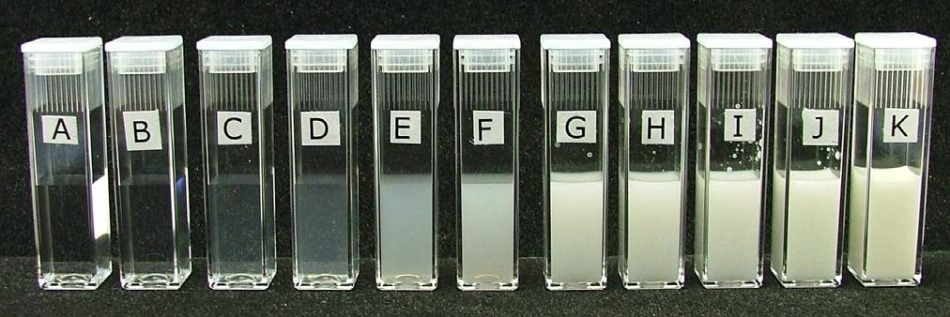Figure 4. Photograph showing the various concentrations of Intralipid that were used in figure 2. The concentrations of the Intralipid samples were A = 0.0001% w/v, B = 0.001% w/v, C = 0.005% w/v, D = 0.01% w/v, E = 0.05% w/v, F = 0.1% w/v, G = 0.5% w/v, H = 0.75% w/v, I = 1% w/v, J = 2% w/v and K = 10% w/v (neat sample). The zeta potentials of samples A to I were successfully measured in the Nano i.e. up to 1% w/v concentration.

The next section describes what effect do inappropriate sample concentrations have on the quality of the data obtained from the measurements.

Table 2. The Intralipid concentrations of the samples contained in the various cuvettes shown in figure 4

Cuvette Label Intralipid Concentration (% w/v)
A 0.0001
B 0.001
C 0.005
D 0.01
E 0.05
F 0.1
G 0.5
H 0.75
I 1
J 2
K 10

## Sample Concentration and Data Quality

### Phase Plots

The mean zeta potentials of samples A to I were successfully measured in the Nano, that is, up to a concentration of 1% w/v. The mean zeta potential is determined from phase analysis light scattering and more information about this method can be obtained from the Malvern Instruments website.

In Figure 5, the phase plot obtained from measurements of sample I (1% w/v) is shown, whereas the phase plot from sample J (2% w/v) is shown in Figure 6. The attenuator indices automatically selected for both these samples were 11 (100% transmission) denoting very low intensities of scattered light detected. On the other hand, while the phase plot obtained with sample I is excellent, the data obtained for sample J is very poor.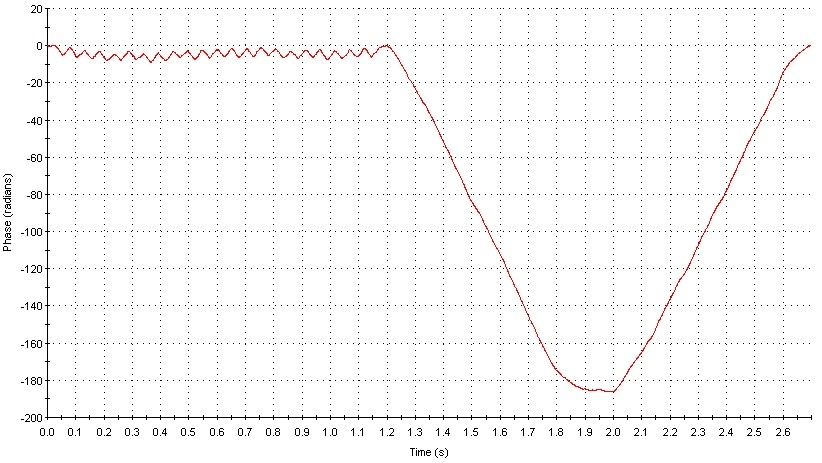Figure 5. Phase plot obtained from measurement of sample I (1% w/v).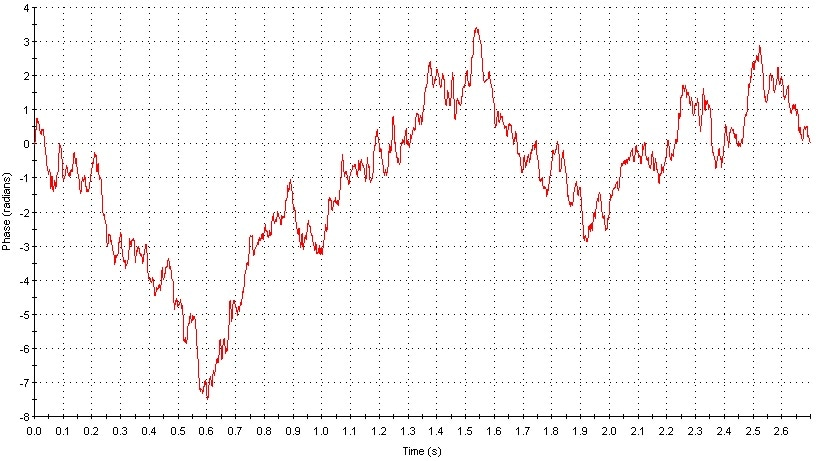Figure 6. Phase plot obtained from measurement of sample J (2% w/v).

When the phase plots from both samples are over plotted (Figure 7), the comparison in data quality can be seen very clearly. The upper limit of concentration for reliable mean zeta potential values for this sample is hence 1% w/v.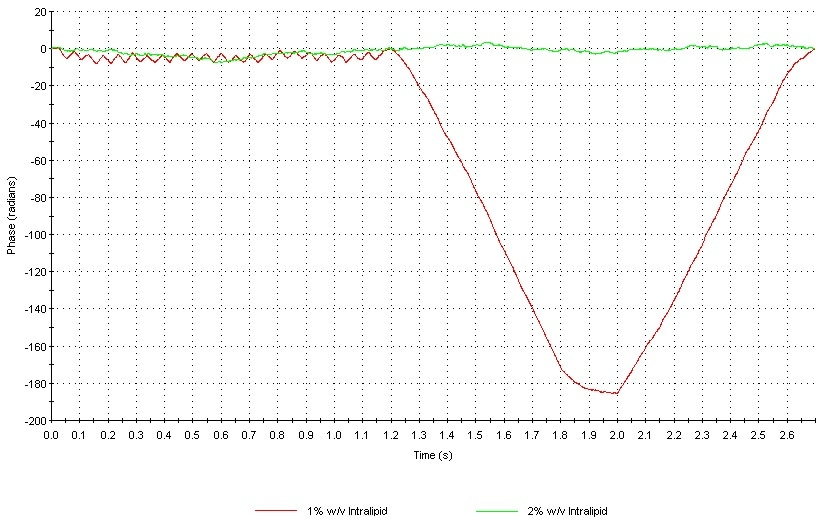Figure 7. Overplot of the phase plots obtained for samples I (1% w/v) and J (2% w/v) illustrating the difference in data quality.

### Frequency Plots

The zeta potential distribution is achieved from the frequency distribution resulting from a Fourier transform during the slow field reversal (SFR) part of the measurement. Although it is possible to measure the mean zeta potential at concentrations of up to 1% w/v, the zeta potential distributions obtained at this concentration are very wide because of the low signal to noise ratio of the detected light. Figure 8 represents an over plot of the frequency distributions obtained for samples I (1% w/v) and G (0.5% w/v), respectively.

For sample 1, the distribution is very wide with the zeta potential deviation of about 80 mV. This is the standard deviation of the peak and indicates the low signal to noise ratio obtained from this measurement. The frequency distribution acquired for sample G (0.5% w/v) has a zeta potential deviation of about 10 mV because of the much narrower distribution width. Hence, the upper limit of concentration for obtaining good zeta potential distributions is 0.5% w/v.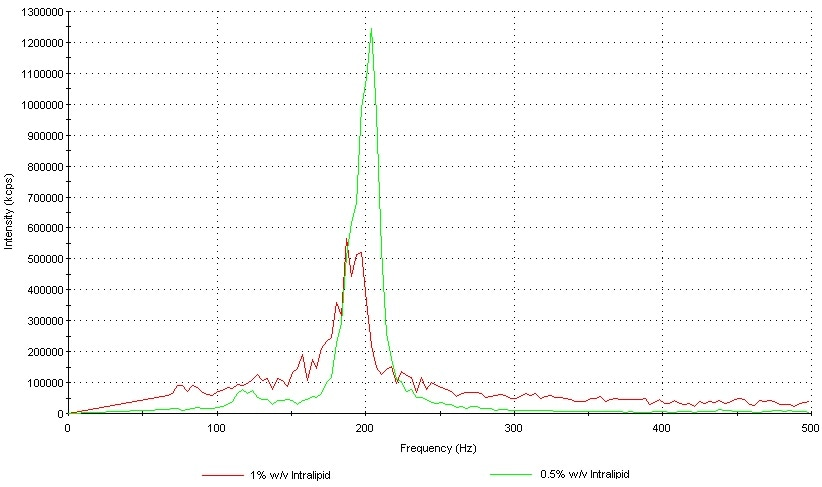Figure 8. Frequency distribution plots obtained from samples I (1% w/v) and G (0.5% w/v) respectively.

## Conclusions

It is not easy to define the upper and lower limits of concentration to successfully measure the zeta potential of a sample. The reason for this is a number of factors will dictate these limits of concentration and these have been discussed in this article.

Eventually, the concentration limits of any sample must be determined experimentally by measuring the sample at various concentrations and viewing the effect on the data quality obtained.

On the whole, the concentration limits for zeta potential can be summarized as follows:

• As the particle size of the sample becomes larger, the minimum and maximum concentrations become lower at which the sample can be successfully measured
• If the particle size of the sample is smaller, the concentration that can be measured is higher
• As the relative refractive index becomes lower, the concentration at which the sample can be successfully measured becomes higher

It is expected that the case study of Intralipid discussed here offers guidance as to the limits of concentration that can be measured in a Zetasizer Nano.

Finally, the quality of the frequency plot and phase plot data is analyzed in a zeta potential quality report that is available in version 4.10 of the Nano software.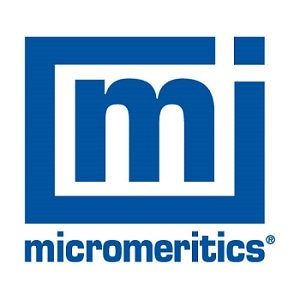This information has been sourced, reviewed and adapted from materials provided by Micromeritics Instrument Corporation.

## Citations

• APA

Micromeritics Instrument Corporation. (2019, December 09). Limits of Concentrations when Measuring Zeta Potential. AZoM. Retrieved on October 20, 2020 from https://www.azom.com/article.aspx?ArticleID=16795.

• MLA

Micromeritics Instrument Corporation. "Limits of Concentrations when Measuring Zeta Potential". AZoM. 20 October 2020. <https://www.azom.com/article.aspx?ArticleID=16795>.

• Chicago

Micromeritics Instrument Corporation. "Limits of Concentrations when Measuring Zeta Potential". AZoM. https://www.azom.com/article.aspx?ArticleID=16795. (accessed October 20, 2020).

• Harvard

Micromeritics Instrument Corporation. 2019. Limits of Concentrations when Measuring Zeta Potential. AZoM, viewed 20 October 2020, https://www.azom.com/article.aspx?ArticleID=16795.